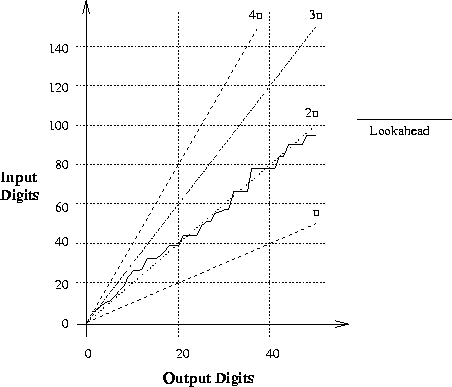Next: Dyadic digit swell Up: Lookahead Results Previous: Signed Binary Multiplication

### Division

The division a/b shows a lookahead of roughly 2n (see figure 7.3). This is above the minimum possible (n), but well below the predicted maximum 4n. Other experiments show this to be fairly typical behaviour unless the numerator and denominator are equal, in which case a lookahead of n will occur.

This experiment avoids the constant factor in the lookahead due to modification of the denominator mantissa and conversion back from dyadic and signed binary digits. In general a lookahead of (2n+c) for some constant c appears probable using the division algorithm.

Note that the analysis given in this section is based upon the rate that the algorithm consumes digits from the input. It is possible that jumps in the algorithm steeper than the 4n predicted maximum may occur if part of the algorithm examines more digits without consuming them (and indeed this can be observed in figure 7.3). However this will never add more than a small constant to the lookahead and digits cannot be consumed faster than 4n.Next: Dyadic digit swell Up: Lookahead Results Previous: Signed Binary Multiplication
Martin Escardo
5/11/2000